# Solutions_to_Chapters_7_and_8_Problem_Sets.ppt

1 de Jun de 2023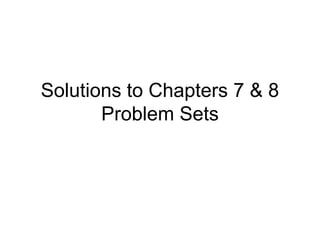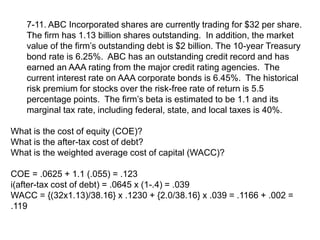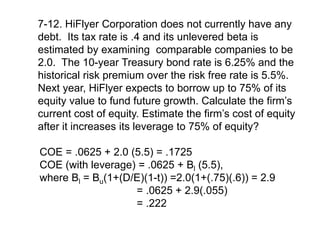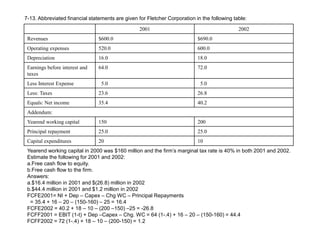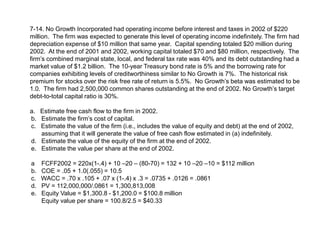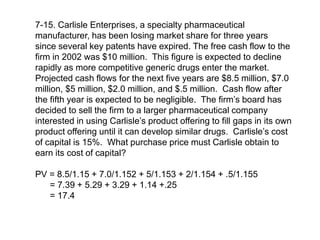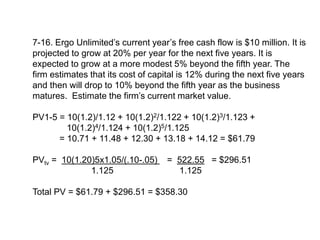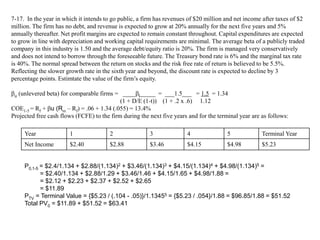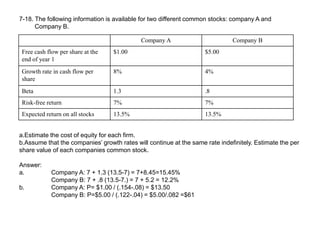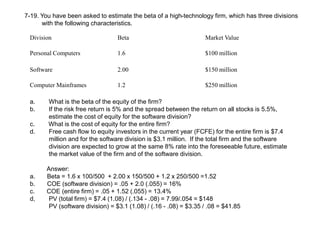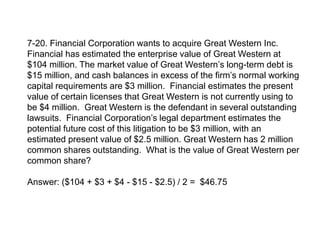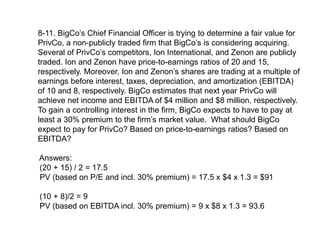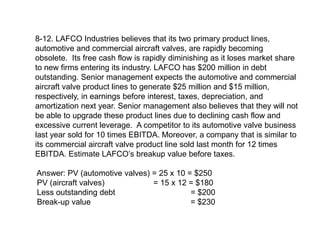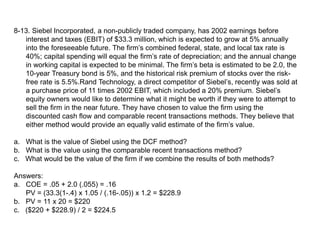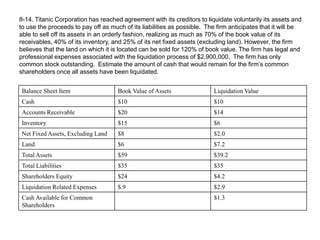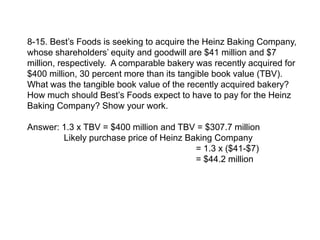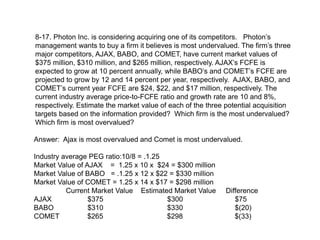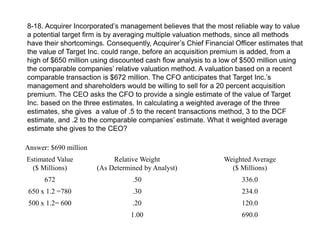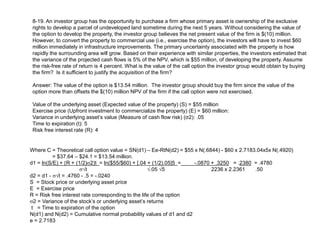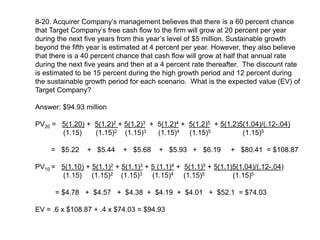1 de 21

### Solutions_to_Chapters_7_and_8_Problem_Sets.ppt

• 1. Solutions to Chapters 7 & 8 Problem Sets
• 2. 7-11. ABC Incorporated shares are currently trading for \$32 per share. The firm has 1.13 billion shares outstanding. In addition, the market value of the firm’s outstanding debt is \$2 billion. The 10-year Treasury bond rate is 6.25%. ABC has an outstanding credit record and has earned an AAA rating from the major credit rating agencies. The current interest rate on AAA corporate bonds is 6.45%. The historical risk premium for stocks over the risk-free rate of return is 5.5 percentage points. The firm’s beta is estimated to be 1.1 and its marginal tax rate, including federal, state, and local taxes is 40%. What is the cost of equity (COE)? What is the after-tax cost of debt? What is the weighted average cost of capital (WACC)? COE = .0625 + 1.1 (.055) = .123 i(after-tax cost of debt) = .0645 x (1-.4) = .039 WACC = {(32x1.13)/38.16} x .1230 + {2.0/38.16} x .039 = .1166 + .002 = .119
• 3. 7-12. HiFlyer Corporation does not currently have any debt. Its tax rate is .4 and its unlevered beta is estimated by examining comparable companies to be 2.0. The 10-year Treasury bond rate is 6.25% and the historical risk premium over the risk free rate is 5.5%. Next year, HiFlyer expects to borrow up to 75% of its equity value to fund future growth. Calculate the firm’s current cost of equity. Estimate the firm’s cost of equity after it increases its leverage to 75% of equity? COE = .0625 + 2.0 (5.5) = .1725 COE (with leverage) = .0625 + Bl (5.5), where Bl = Bu(1+(D/E)(1-t)) =2.0(1+(.75)(.6)) = 2.9 = .0625 + 2.9(.055) = .222
• 4. 7-13. Abbreviated financial statements are given for Fletcher Corporation in the following table: 2001 2002 Revenues \$600.0 \$690.0 Operating expenses 520.0 600.0 Depreciation 16.0 18.0 Earnings before interest and taxes 64.0 72.0 Less Interest Expense 5.0 5.0 Less: Taxes 23.6 26.8 Equals: Net income 35.4 40.2 Addendum: Yearend working capital 150 200 Principal repayment 25.0 25.0 Capital expenditures 20 10 Yearend working capital in 2000 was \$160 million and the firm’s marginal tax rate is 40% in both 2001 and 2002. Estimate the following for 2001 and 2002: a.Free cash flow to equity. b.Free cash flow to the firm. Answers: a.\$16.4 million in 2001 and \$(26.8) million in 2002 b.\$44.4 million in 2001 and \$1.2 million in 2002 FCFE2001= NI + Dep – Capex – Chg WC – Principal Repayments = 35.4 + 16 – 20 – (150-160) – 25 = 16.4 FCFE2002 = 40.2 + 18 – 10 – (200 –150) –25 = -26.8 FCFF2001 = EBIT (1-t) + Dep –Capex – Chg. WC = 64 (1-.4) + 16 – 20 – (150-160) = 44.4 FCFF2002 = 72 (1-.4) + 18 – 10 – (200-150) = 1.2
• 5. 7-14. No Growth Incorporated had operating income before interest and taxes in 2002 of \$220 million. The firm was expected to generate this level of operating income indefinitely. The firm had depreciation expense of \$10 million that same year. Capital spending totaled \$20 million during 2002. At the end of 2001 and 2002, working capital totaled \$70 and \$80 million, respectively. The firm’s combined marginal state, local, and federal tax rate was 40% and its debt outstanding had a market value of \$1.2 billion. The 10-year Treasury bond rate is 5% and the borrowing rate for companies exhibiting levels of creditworthiness similar to No Growth is 7%. The historical risk premium for stocks over the risk free rate of return is 5.5%. No Growth’s beta was estimated to be 1.0. The firm had 2,500,000 common shares outstanding at the end of 2002. No Growth’s target debt-to-total capital ratio is 30%. a. Estimate free cash flow to the firm in 2002. b. Estimate the firm’s cost of capital. c. Estimate the value of the firm (i.e., includes the value of equity and debt) at the end of 2002, assuming that it will generate the value of free cash flow estimated in (a) indefinitely. d. Estimate the value of the equity of the firm at the end of 2002. e. Estimate the value per share at the end of 2002. a FCFF2002 = 220x(1-.4) + 10 –20 – (80-70) = 132 + 10 –20 –10 = \$112 million b. COE = .05 + 1.0(.055) = 10.5 c. WACC = .70 x .105 + .07 x (1-.4) x .3 = .0735 + .0126 = .0861 d. PV = 112,000,000/.0861 = 1,300,813,008 e. Equity Value = \$1,300.8 - \$1,200.0 = \$100.8 million Equity value per share = 100.8/2.5 = \$40.33
• 6. 7-15. Carlisle Enterprises, a specialty pharmaceutical manufacturer, has been losing market share for three years since several key patents have expired. The free cash flow to the firm in 2002 was \$10 million. This figure is expected to decline rapidly as more competitive generic drugs enter the market. Projected cash flows for the next five years are \$8.5 million, \$7.0 million, \$5 million, \$2.0 million, and \$.5 million. Cash flow after the fifth year is expected to be negligible. The firm’s board has decided to sell the firm to a larger pharmaceutical company interested in using Carlisle’s product offering to fill gaps in its own product offering until it can develop similar drugs. Carlisle’s cost of capital is 15%. What purchase price must Carlisle obtain to earn its cost of capital? PV = 8.5/1.15 + 7.0/1.152 + 5/1.153 + 2/1.154 + .5/1.155 = 7.39 + 5.29 + 3.29 + 1.14 +.25 = 17.4
• 7. 7-16. Ergo Unlimited’s current year’s free cash flow is \$10 million. It is projected to grow at 20% per year for the next five years. It is expected to grow at a more modest 5% beyond the fifth year. The firm estimates that its cost of capital is 12% during the next five years and then will drop to 10% beyond the fifth year as the business matures. Estimate the firm’s current market value. PV1-5 = 10(1.2)/1.12 + 10(1.2)2/1.122 + 10(1.2)3/1.123 + 10(1.2)4/1.124 + 10(1.2)5/1.125 = 10.71 + 11.48 + 12.30 + 13.18 + 14.12 = \$61.79 PVtv = 10(1.20)5x1.05/(.10-.05) = 522.55 = \$296.51 1.125 1.125 Total PV = \$61.79 + \$296.51 = \$358.30
• 8. 7-17. In the year in which it intends to go public, a firm has revenues of \$20 million and net income after taxes of \$2 million. The firm has no debt, and revenue is expected to grow at 20% annually for the next five years and 5% annually thereafter. Net profit margins are expected to remain constant throughout. Capital expenditures are expected to grow in line with depreciation and working capital requirements are minimal. The average beta of a publicly traded company in this industry is 1.50 and the average debt/equity ratio is 20%. The firm is managed very conservatively and does not intend to borrow through the foreseeable future. The Treasury bond rate is 6% and the marginal tax rate is 40%. The normal spread between the return on stocks and the risk free rate of return is believed to be 5.5%. Reflecting the slower growth rate in the sixth year and beyond, the discount rate is expected to decline by 3 percentage points. Estimtate the value of the firm’s equity. u (unlevered beta) for comparable firms = ____l_____ = ___1.5___ = 1.5 = 1.34 (1 + D/E (1-t)) (1 + .2 x .6) 1.12 COE1-5 = Rf + u (Rm – Rf) = .06 + 1.34 (.055) = 13.4% Projected free cash flows (FCFE) to the firm during the next five years and for the terminal year are as follows: Year 1 2 3 4 5 Terminal Year Net Income \$2.40 \$2.88 \$3.46 \$4.15 \$4.98 \$5.23 P0,1-5 = \$2.4/1.134 + \$2.88/(1.134)2 + \$3.46/(1.134)3 + \$4.15/(1.134)4 + \$4.98/(1.134)5 = = \$2.40/1.134 + \$2.88/1.29 + \$3.46/1.46 + \$4.15/1.65 + \$4.98/1.88 = = \$2.12 + \$2.23 + \$2.37 + \$2.52 + \$2.65 = \$11.89 PTV = Terminal Value = {\$5.23 / (.104 - .05)}/1.13455 = {\$5.23 / .054}/1.88 = \$96.85/1.88 = \$51.52 Total PV0 = \$11.89 + \$51.52 = \$63.41
• 9. 7-18. The following information is available for two different common stocks: company A and Company B. Company A Company B Free cash flow per share at the end of year 1 \$1.00 \$5.00 Growth rate in cash flow per share 8% 4% Beta 1.3 .8 Risk-free return 7% 7% Expected return on all stocks 13.5% 13.5% a.Estimate the cost of equity for each firm. b.Assume that the companies’ growth rates will continue at the same rate indefinitely. Estimate the per share value of each companies common stock. Answer: a. Company A: 7 + 1.3 (13.5-7) = 7+8.45=15.45% Company B: 7 + .8 (13.5-7.) = 7 + 5.2 = 12.2% b. Company A: P= \$1.00 / (.154-.08) = \$13.50 Company B: P=\$5.00 / (.122-.04) = \$5.00/.082 =\$61
• 10. 7-19. You have been asked to estimate the beta of a high-technology firm, which has three divisions with the following characteristics. Division Beta Market Value Personal Computers 1.6 \$100 million Software 2.00 \$150 million Computer Mainframes 1.2 \$250 million a. What is the beta of the equity of the firm? b. If the risk free return is 5% and the spread between the return on all stocks is 5.5%, estimate the cost of equity for the software division? c. What is the cost of equity for the entire firm? d. Free cash flow to equity investors in the current year (FCFE) for the entire firm is \$7.4 million and for the software division is \$3.1 million. If the total firm and the software division are expected to grow at the same 8% rate into the foreseeable future, estimate the market value of the firm and of the software division. Answer: a. Beta = 1.6 x 100/500 + 2.00 x 150/500 + 1.2 x 250/500 =1.52 b. COE (software division) = .05 + 2.0 (.055) = 16% c. COE (entire firm) = .05 + 1.52 (.055) = 13.4% d, PV (total firm) = \$7.4 (1.08) / (.134 - .08) = 7.99/.054 = \$148 PV (software division) = \$3.1 (1.08) / (.16 - .08) = \$3.35 / .08 = \$41.85
• 11. 7-20. Financial Corporation wants to acquire Great Western Inc. Financial has estimated the enterprise value of Great Western at \$104 million. The market value of Great Western’s long-term debt is \$15 million, and cash balances in excess of the firm’s normal working capital requirements are \$3 million. Financial estimates the present value of certain licenses that Great Western is not currently using to be \$4 million. Great Western is the defendant in several outstanding lawsuits. Financial Corporation’s legal department estimates the potential future cost of this litigation to be \$3 million, with an estimated present value of \$2.5 million. Great Western has 2 million common shares outstanding. What is the value of Great Western per common share? Answer: (\$104 + \$3 + \$4 - \$15 - \$2.5) / 2 = \$46.75
• 12. 8-11. BigCo’s Chief Financial Officer is trying to determine a fair value for PrivCo, a non-publicly traded firm that BigCo’s is considering acquiring. Several of PrivCo’s competitors, Ion International, and Zenon are publicly traded. Ion and Zenon have price-to-earnings ratios of 20 and 15, respectively. Moreover, Ion and Zenon’s shares are trading at a multiple of earnings before interest, taxes, depreciation, and amortization (EBITDA) of 10 and 8, respectively. BigCo estimates that next year PrivCo will achieve net income and EBITDA of \$4 million and \$8 million, respectively. To gain a controlling interest in the firm, BigCo expects to have to pay at least a 30% premium to the firm’s market value. What should BigCo expect to pay for PrivCo? Based on price-to-earnings ratios? Based on EBITDA? Answers: (20 + 15) / 2 = 17.5 PV (based on P/E and incl. 30% premium) = 17.5 x \$4 x 1.3 = \$91 (10 + 8)/2 = 9 PV (based on EBITDA incl. 30% premium) = 9 x \$8 x 1.3 = 93.6
• 13. 8-12. LAFCO Industries believes that its two primary product lines, automotive and commercial aircraft valves, are rapidly becoming obsolete. Its free cash flow is rapidly diminishing as it loses market share to new firms entering its industry. LAFCO has \$200 million in debt outstanding. Senior management expects the automotive and commercial aircraft valve product lines to generate \$25 million and \$15 million, respectively, in earnings before interest, taxes, depreciation, and amortization next year. Senior management also believes that they will not be able to upgrade these product lines due to declining cash flow and excessive current leverage. A competitor to its automotive valve business last year sold for 10 times EBITDA. Moreover, a company that is similar to its commercial aircraft valve product line sold last month for 12 times EBITDA. Estimate LAFCO’s breakup value before taxes. Answer: PV (automotive valves) = 25 x 10 = \$250 PV (aircraft valves) = 15 x 12 = \$180 Less outstanding debt = \$200 Break-up value = \$230
• 14. 8-13. Siebel Incorporated, a non-publicly traded company, has 2002 earnings before interest and taxes (EBIT) of \$33.3 million, which is expected to grow at 5% annually into the foreseeable future. The firm’s combined federal, state, and local tax rate is 40%; capital spending will equal the firm’s rate of depreciation; and the annual change in working capital is expected to be minimal. The firm’s beta is estimated to be 2.0, the 10-year Treasury bond is 5%, and the historical risk premium of stocks over the risk- free rate is 5.5%.Rand Technology, a direct competitor of Siebel’s, recently was sold at a purchase price of 11 times 2002 EBIT, which included a 20% premium. Siebel’s equity owners would like to determine what it might be worth if they were to attempt to sell the firm in the near future. They have chosen to value the firm using the discounted cash flow and comparable recent transactions methods. They believe that either method would provide an equally valid estimate of the firm’s value. a. What is the value of Siebel using the DCF method? b. What is the value using the comparable recent transactions method? c. What would be the value of the firm if we combine the results of both methods? Answers: a. COE = .05 + 2.0 (.055) = .16 PV = (33.3(1-.4) x 1.05 / (.16-.05)) x 1.2 = \$228.9 b. PV = 11 x 20 = \$220 c. (\$220 + \$228.9) / 2 = \$224.5
• 15. 8-14. Titanic Corporation has reached agreement with its creditors to liquidate voluntarily its assets and to use the proceeds to pay off as much of its liabilities as possible. The firm anticipates that it will be able to sell off its assets in an orderly fashion, realizing as much as 70% of the book value of its receivables, 40% of its inventory, and 25% of its net fixed assets (excluding land). However, the firm believes that the land on which it is located can be sold for 120% of book value. The firm has legal and professional expenses associated with the liquidation process of \$2,900,000. The firm has only common stock outstanding. Estimate the amount of cash that would remain for the firm’s common shareholders once all assets have been liquidated. Balance Sheet Item Book Value of Assets Liquidation Value Cash \$10 \$10 Accounts Receivable \$20 \$14 Inventory \$15 \$6 Net Fixed Assets, Excluding Land \$8 \$2.0 Land \$6 \$7.2 Total Assets \$59 \$39.2 Total Liabilities \$35 \$35 Shareholders Equity \$24 \$4.2 Liquidation Related Expenses \$.9 \$2.9 Cash Available for Common Shareholders \$1.3
• 16. 8-15. Best’s Foods is seeking to acquire the Heinz Baking Company, whose shareholders’ equity and goodwill are \$41 million and \$7 million, respectively. A comparable bakery was recently acquired for \$400 million, 30 percent more than its tangible book value (TBV). What was the tangible book value of the recently acquired bakery? How much should Best’s Foods expect to have to pay for the Heinz Baking Company? Show your work. Answer: 1.3 x TBV = \$400 million and TBV = \$307.7 million Likely purchase price of Heinz Baking Company = 1.3 x (\$41-\$7) = \$44.2 million
• 17. 8-16. Delhi Automotive Inc. is the leading supplier of specialty fasteners for passenger cars in the U.S. market, with an estimated 25 percent share of this \$5 billion market. Delhi’s rapid growth in recent years has been fueled by high levels of reinvestment in the firm. While this has resulted in the firm having “state of the art” plants, it has also resulted in the firm showing limited profitability and positive cash flow. Delhi is privately owned and has announced that it is going to undertake an initial public offering in the near future. Investors know that economies of scale are important in this high fixed cost industry and understand that market share is an important determinant of future profitability. Thornton Auto Inc., a publicly traded firm and the leader in this market, has an estimated market share of 38 percent and an \$800 million market value. How should investors value the Delhi IPO? Answer: If the market leader has a market value and market share of \$800 million and 38%, respectively, the market is valuing each percentage point of market share at \$21.05 million (i.e., \$800 million/38). If Delhi has a market share of 25 percent, the IPO could have a potential value of \$526.3 million (i.e., 25 points of market share times \$21.05 million).
• 18. 8-17. Photon Inc. is considering acquiring one of its competitors. Photon’s management wants to buy a firm it believes is most undervalued. The firm’s three major competitors, AJAX, BABO, and COMET, have current market values of \$375 million, \$310 million, and \$265 million, respectively. AJAX’s FCFE is expected to grow at 10 percent annually, while BABO’s and COMET’s FCFE are projected to grow by 12 and 14 percent per year, respectively. AJAX, BABO, and COMET’s current year FCFE are \$24, \$22, and \$17 million, respectively. The current industry average price-to-FCFE ratio and growth rate are 10 and 8%, respectively. Estimate the market value of each of the three potential acquisition targets based on the information provided? Which firm is the most undervalued? Which firm is most overvalued? Answer: Ajax is most overvalued and Comet is most undervalued. Industry average PEG ratio:10/8 = .1.25 Market Value of AJAX = 1.25 x 10 x \$24 = \$300 million Market Value of BABO = .1.25 x 12 x \$22 = \$330 million Market Value of COMET = 1.25 x 14 x \$17 = \$298 million Current Market Value Estimated Market Value Difference AJAX \$375 \$300 \$75 BABO \$310 \$330 \$(20) COMET \$265 \$298 \$(33)
• 19. 8-18. Acquirer Incorporated’s management believes that the most reliable way to value a potential target firm is by averaging multiple valuation methods, since all methods have their shortcomings. Consequently, Acquirer’s Chief Financial Officer estimates that the value of Target Inc. could range, before an acquisition premium is added, from a high of \$650 million using discounted cash flow analysis to a low of \$500 million using the comparable companies’ relative valuation method. A valuation based on a recent comparable transaction is \$672 million. The CFO anticipates that Target Inc.’s management and shareholders would be willing to sell for a 20 percent acquisition premium. The CEO asks the CFO to provide a single estimate of the value of Target Inc. based on the three estimates. In calculating a weighted average of the three estimates, she gives a value of .5 to the recent transactions method, 3 to the DCF estimate, and .2 to the comparable companies’ estimate. What it weighted average estimate she gives to the CEO? Answer: \$690 million Estimated Value (\$ Millions) Relative Weight (As Determined by Analyst) Weighted Average (\$ Millions) 672 .50 336.0 650 x 1.2 =780 .30 234.0 500 x 1.2= 600 .20 120.0 1.00 690.0
• 20. 8-19. An investor group has the opportunity to purchase a firm whose primary asset is ownership of the exclusive rights to develop a parcel of undeveloped land sometime during the next 5 years. Without considering the value of the option to develop the property, the investor group believes the net present value of the firm is \$(10) million. However, to convert the property to commercial use (i.e., exercise the option), the investors will have to invest \$60 million immediately in infrastructure improvements. The primary uncertainty associated with the property is how rapidly the surrounding area will grow. Based on their experience with similar properties, the investors estimated that the variance of the projected cash flows is 5% of the NPV, which is \$55 million, of developing the property. Assume the risk-free rate of return is 4 percent. What is the value of the call option the investor group would obtain by buying the firm? Is it sufficient to justify the acquisition of the firm? Answer: The value of the option is \$13.54 million. The investor group should buy the firm since the value of the option more than offsets the \$(10) million NPV of the firm if the call option were not exercised. Value of the underlying asset (Expected value of the property) (S) = \$55 million Exercise price (Upfront investment to commercialize the property) (E) = \$60 million: Variance in underlying asset’s value (Measure of cash flow risk) (σ2): .05 Time to expiration (t): 5 Risk free interest rate (R): 4 Where C = Theoretical call option value = SN(d1) – Ee-RtN(d2) = \$55 x N(.6844) - \$60 x 2.7183.04x5x N(.4920) = \$37.64 – \$24.1 = \$13.54 million. d1 = ln(S/E) + R + (1/2)2t = ln(\$55/\$60) + [.04 + (1/2).05]5 = -.0870 + .3250 = .2380 = .4780 t .05 5 2236 x 2.2361 .50 d2 = d1 - t = .4760 - .5 = -.0240 S = Stock price or underlying asset price E = Exercise price R = Risk free interest rate corresponding to the life of the option 2 = Variance of the stock’s or underlying asset’s returns t = Time to expiration of the option N(d1) and N(d2) = Cumulative normal probability values of d1 and d2 e = 2.7183
• 21. 8-20. Acquirer Company’s management believes that there is a 60 percent chance that Target Company’s free cash flow to the firm will grow at 20 percent per year during the next five years from this year’s level of \$5 million. Sustainable growth beyond the fifth year is estimated at 4 percent per year. However, they also believe that there is a 40 percent chance that cash flow will grow at half that annual rate during the next five years and then at a 4 percent rate thereafter. The discount rate is estimated to be 15 percent during the high growth period and 12 percent during the sustainable growth period for each scenario. What is the expected value (EV) of Target Company? Answer: \$94.93 million PV20 = 5(1.20) + 5(1.2)2 + 5(1.2)3 + 5(1.2)4 + 5(1.2)5 + 5(1.2)5(1.04)/(.12-.04) (1.15) (1.15)2 (1.15)3 (1.15)4 (1.15)5 (1.15)5 = \$5.22 + \$5.44 + \$5.68 + \$5.93 + \$6.19 + \$80.41 = \$108.87 PV10 = 5(1.10) + 5(1.1)2 + 5(1.1)3 + 5 (1.1)4 + 5(1.1)5 + 5(1.1)5(1.04)/(.12-.04) (1.15) (1.15)2 (1.15)3 (1.15)4 (1.15)5 (1.15)5 = \$4.78 + \$4.57 + \$4.38 + \$4.19 + \$4.01 + \$52.1 = \$74.03 EV = .6 x \$108.87 + .4 x \$74.03 = \$94.93